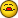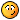# C++ question

W

#### web1110

Hi y'all,

This is the wrong venue, but I have C++ question.

I was playing with VS 6.0 and tried this program:

#include "stdafx.h"
#include <iostream.h>

class prop
{
private:
int x;
int y;

public:
prop(int x, int y);
int& X();
int& operator= (int);
};

prop:rop(int x, int y)
{
prop::x=x;
prop::y=y;
}

int& prop::X()
{
return x;
}

int& prop:perator=(int=0)
{
return x;
}

int main(int argc, char* argv[])
{
prop a(1, 2);
cout << "a.X=" << a.X() << '\n';
a.X=10; <-----------------------------------
cout << "a.X=" << a.X() << '\n';
return 0;
}

The marked line produces the error:

S:\tmp\VS60\Props\Props.cpp(39) : error C2659: '=' : overloaded function
as left operand

Can someone explain why?

Thanx,
Bill

A

#### Alex Passos

I don't believe that

int& operator= (int);

has any returns.

void operator=(const int& x) {
somevalue = x;
}

Alex

A

#### Angel J. Hernández M.

I can see you're overloading the = operator however when you try to assign a
value to X (class member) you get the following error because X is a member
function it returns some address (in your case the private field, x).

Regards,

C

#### Claudio Grazioli

Hi y'all,

This is the wrong venue, but I have C++ question.

I was playing with VS 6.0 and tried this program:

#include "stdafx.h"
#include <iostream.h>

class prop
{
private:
int x;
int y;

public:
prop(int x, int y);
int& X();
int& operator= (int);
};

prop:rop(int x, int y)
{
prop::x=x;
prop::y=y;
}

int& prop::X()
{
return x;
}

int& prop:perator=(int=0)
{
return x;
}

int main(int argc, char* argv[])
{
prop a(1, 2);
cout << "a.X=" << a.X() << '\n';
a.X=10; <-----------------------------------
cout << "a.X=" << a.X() << '\n';
return 0;
}

The marked line produces the error:

S:\tmp\VS60\Props\Props.cpp(39) : error C2659: '=' : overloaded function
as left operand

Can someone explain why?

Thanx,
Bill

a.X=10

X is a method of prop. So you're trying to assign 10 to the method X. Of
course, that's not possible.

A

#### Alex Passos

a = 10 to use the overloaded "=".

Alex

Claudio Grazioli said:
Hi y'all,

This is the wrong venue, but I have C++ question.

I was playing with VS 6.0 and tried this program:

#include "stdafx.h"
#include <iostream.h>

class prop
{
private:
int x;
int y;

public:
prop(int x, int y);
int& X();
int& operator= (int);
};

prop:rop(int x, int y)
{
prop::x=x;
prop::y=y;
}

int& prop::X()
{
return x;
}

int& prop:perator=(int=0)
{
return x;
}

int main(int argc, char* argv[])
{
prop a(1, 2);
cout << "a.X=" << a.X() << '\n';
a.X=10; <-----------------------------------
cout << "a.X=" << a.X() << '\n';
return 0;
}

The marked line produces the error:

S:\tmp\VS60\Props\Props.cpp(39) : error C2659: '=' : overloaded
function
as left operand

Can someone explain why?

Thanx,
Bill

a.X=10

X is a method of prop. So you're trying to assign 10 to the method X. Of
course, that's not possible.

J

#### James Curran

No. Operator=() intricsically returns the right-hand side. So things
like a=b=c=d=e=0 are allowed.

J

#### James Curran

You can't take emulating a property quite that far(*). X is still a
method, and must be called as X():

a.X() = 10;

(*) Actually, you can get but it's way more work than you want to do.
Define X as a public data member of type PropInt. Put you setter in the
PropInt:ropInt(int) constructor, and the getter in PropInt:perator
int().